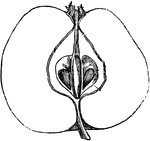### Apple

"Perpendicular section of the fruit of Pyrus malus." -Lindley, 1853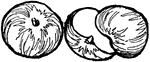### Apples

Two apples. One is whole and the other is split in half.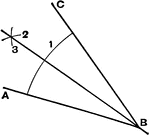### Bisect An Angle

Method to bisect an angle### Half-Mourning Butterfly

"The Galatea Butterfly, P. Galatea, A Euoprean species, called The Half-Mourning Butterfly,…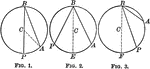### Circles With Inscribed Angles

Illustration of equal circles to show that an inscribed angle is measured by half the arc intercepted…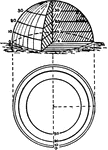### Contours of a half sphere

"The contours of a half sphere are a series of circles, far apart near the center (top) and near together…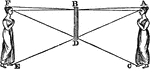### Mirror Half the Length of the Object

"This will be understood [here] where the ray of light A B, proceeding from the eye, falls perpendicularly…### Party Per Pale

"Parted per pale, gules and argent. PARTY PER PALE. This signifies a shield parted by a perpendicular…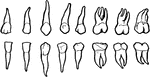### Half set of permanent teeth

"One half of the permanent set of teeth." — Ritchie, 1918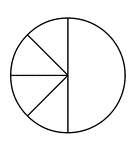### Four Eighths and One Half of a Pie Fraction

A circle subdivided into four eighths and one half.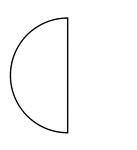### Half of a Fraction Pie

One half of a circle (vertical left).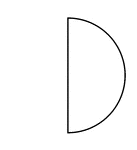### Half of a Fraction Pie

One half of a circle (vertical right).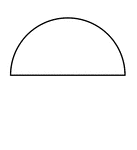### Half of a Fraction Pie

One half of a circle (horizontal top).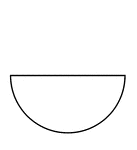### Half of a Fraction Pie

One half of a circle (horizontal bottom).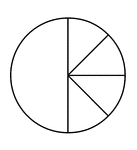### One Half and Four Eighths of a Pie Fraction

A circle subdivided into one half and four eighths.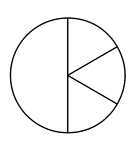### One Half and Three Sixths of a Pie Fraction

A circle subdivided into one half and three sixths.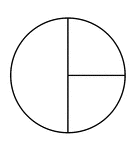### One Half and Two Quarters of a Pie Fraction

A circle subdivided into one half and two quarters.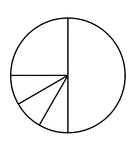### One Quarter, Three Twelfths, and One Half of a Pie Fraction

A circle subdivided into one quarter, three twelfths, and one half.### One Quarter, Two Eighths, and One Half of a Pie Fraction

A circle subdivided into one quarter, two eighths, and one half.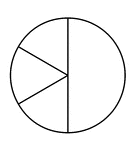### Three Sixths and One Half of a Pie Fraction

A circle subdivided into three sixths and one half.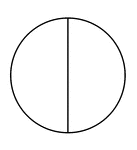### Two Halves of a Fraction Pie

A circle divided in half vertically.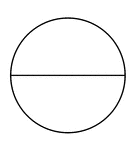### Two Halves of a Fraction Pie

A circle divided in half horizontally.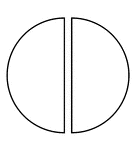### Two Halves of a Fraction Pie

A circle divided in half vertically separated.### Two Halves of a Fraction Pie

A circle divided in half horizontally separated.### Two Quarters and One Half of a Pie Fraction

A circle subdivided into two quarters and one half.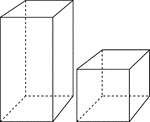### 2 Rectangular Prisms

Illustration of 2 right rectangular prisms. The bases are congruent, but the height of the smaller prism…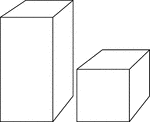### 2 Rectangular Prisms

Illustration of 2 right rectangular prisms. The bases are congruent, but the height of the smaller prism…### Bottom Half of a Regular Dodecahedron

Illustration of a the bottom half of a regular dodecahedron. A regular dodecahedron is a polyhedron…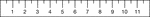### Half Inch Ruler

English ruler with half inch markings.